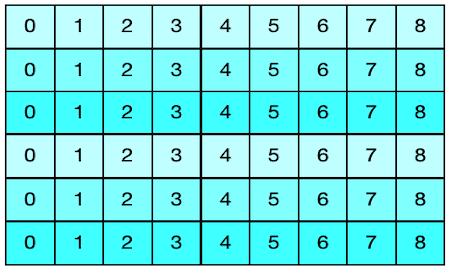# Three effective ways to create modulo n integer sequences.This post compare different ways of generating integer sequences.

A modulo n integer sequence is just a (potentially infinite) integer sequence that wrap to 0 when the current value is n. For example, $0,1,2,3,4,0,1,2,3,4,...$ is a modulo 4 integer sequence.

Modulo-n integer sequences are widely used in programming. For instance, they are necessary in any array-backed data structure (e.g. ring buffers or fixed size queues) in order to reuse elements when the end of the array is reached.

In this post, we will shortly review three different ways to create such sequences examining their pros and cons.

# Integer modulo operator

Let's assume that the current value of the sequence is stored in an integer variable called counter. The traditional way to generate the next value is to increase counter, divide the result by n and then keep the remainder using the modulo operator.

cnt = (cnt + 1) % n;


This solution is easy and readable, but not so performant. Indeed, the integer modulo operator is expensive and requires far more clock cycles than other operations like addition or subtraction.

# Comparison

A second way is to replace the modulo operator with a comparison. We reset counter to 0 when it is equal to n and we increment counter otherwise.

  cnt = (cnt + 1);
if (cnt >= n) cnt = 0;


This approach is usually more efficient than the previous one even if the performance benefits is completely platform dependent.

# Modulo with bitwise and operator

If n is a power of two (i.e. n = 2m), it is possible to create a modulo-n sequence in a very efficient way using the bitwise and operator.

Under this special condition indeed

cnt = (cnt + 1) % n;


becomes equivalent to

cnt = (cnt + 1) & n;


But why this approach works?

When n = 2m, we have that:

1. the modulo operator takes only the least significant m bits of the counter variable

2. n-1 is a number having the last m bits equal to one (i.e. n = 64 = 1000000 in binary, n-1 = 63 = 111111)

So if you consider the bitwise and table of truth, it becomes clear how the bitwise and operator can be used to implement the modulo operator in this particular case.

This third method is very efficient because the bitwise and operator is really fast. The downside is that the method is not applicable to all use cases and it requires some binary math knowledge to be understood.

The following example shows how to generate a modulo-64 sequence using the AND operator:

  cnt = (cnt + 1) & 0x3F;  // n = 64, m = 6


# Conclusion

We reviewed three different methods of generating modulo n sequences of integer. I would generally suggest to use one of the first two methods, since they are more readable and appicable without any restriction. Anyway, if you need a very performant code, you could consider also the third option. For example, if you are implementing a circular buffer, you might consider setting its size to a power of two speed up performance on accessing the buffer. Since I used C++ as reference language, in the code snippets the modulo operator has been represented by % and the biwise and operator by &. If you liked this post, follow me on Twitter to get more related content daily!